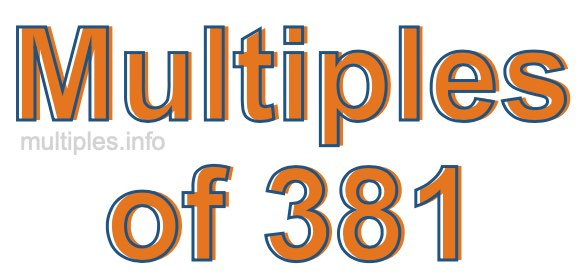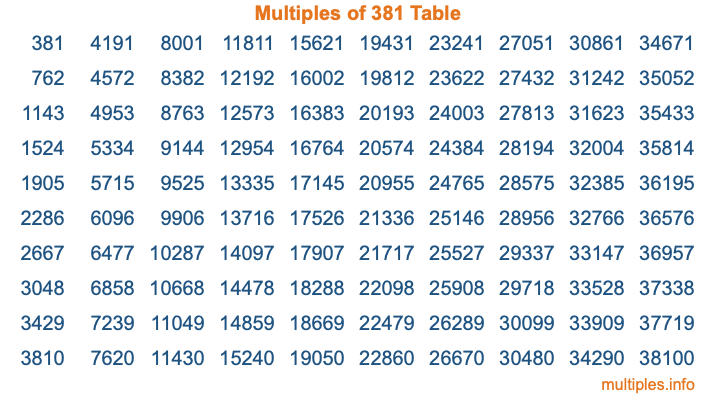Multiples of 381Welcome to the Multiples of 381 page. Here we will first teach you everything you will ever need to know about the multiples of 381, and then give you a study guide summary of everything we taught you to make sure you remember it all. Use this page to look up facts and learn information about the multiples of 381. This page will make you a multiples of three hundred eighty-one expert!

Definition of Multiples of 381
Multiples of 381 are all the numbers that when divided by 381 equal an integer. Each of the multiples of 381 are called a multiple. A multiple of 381 is created by multiplying 381 by an integer.

Therefore, to create a list of multiples of 381, you start with 1 multiplied by 381, then 2 multiplied by 381, then 3 multiplied by 381, and so on for as long as you want. Thus, the list of the first five multiples of 381 is 381, 762, 1143, 1524, and 1905. To see a larger list of multiples of 381, see the printable image of Multiples of 381 further down on this page. We also have a category where you can choose any nth multiple of 381.

Multiples of 381 Checker
The Multiples of 381 Checker below checks to see if any number of your choice is a multiple of 381. In other words, it checks to see if there is any number (integer) that when multiplied by 381 will equal your number. To do that, we divide your number by 381. If the the quotient is an integer, then your number is a multiple of 381.

Is  a multiple of 381?

Least Common Multiple of 381 and ...
A Least Common Multiple (LCM) is the lowest multiple that two or more numbers have in common. This is also called the smallest common multiple or lowest common multiple and is useful to know when you are adding our subtracting fractions. Enter one or more numbers below (381 is already entered) to find the LCM.

Check out our LCM Calculator if you need more details about the Least Common Multiple or if you need the LCM for different numbers for adding and subtraction fractions.

nth Multiple of 381
As we stated above, 381 is the first multiple of 381, 762 is the second multiple of 381, 1143 is the third multiple of 381, and so on. Enter a number below to find the nth multiple of 381.

th multiple of 381

Multiples of 381 vs Factors of 381
381 is a multiple of 381 and a factor of 381, but that is where the similarities end. All postive multiples of 381 are 381 or greater than 381. All positive factors of 381 are 381 or less than 381.

Below is the beginning list of multiples of 381 and the factors of 381 so you can compare:

Multiples of 381: 381, 762, 1143, 1524, 1905, etc.

Factors of 381: 1, 3, 127, 381

As you can see, the multiples of 381 are all the numbers that you can divide by 381 to get a whole number. The factors of 381, on the other hand, are all the whole numbers that you can multiply by another whole number to get 381.

It's also interesting to note that if a number (x) is a factor of 381, then 381 will also be a multiple of that number (x).

Multiples of 381 vs Divisors of 381
The divisors of 381 are all the integers that 381 can be divided by evenly. Below is a list of the divisors of 381.

Divisors of 381: 1, 3, 127, 381

The interesting thing to note here is that if you take any multiple of 381 and divide it by a divisor of 381, you will see that the quotient is an integer.

Multiples of 381 Table
Below is an image of the first 100 multiples of 381 in a table. The table is in chronological order, column by column. The first column has the first ten multiples of 381, the second column has the next ten multiples of 381, and so on.The Multiples of 381 Table is also referred to as the 381 Times Table or Times Table of 381. You are welcome to print out our table for your studies.

Negative Multiples of 381
Although not often discussed or needed in math, it is worth mentioning that you can make a list of negative multiples of 381 by multiplying 381 by -1, then by -2, then by -3, and so on, to get the following list of negative multiples of 381:

-381, -762, -1143, -1524, -1905, etc.

Multiples of 381 Summary
Below is a summary of important Multiples of 381 facts that we have discussed on this page. To retain the knowledge on this page, we recommend that you read through the summary and explain to yourself or a study partner why they hold true.

There are an infinite number of multiples of 381.

A multiple of 381 divided by 381 will equal a whole number.

381 divided by a factor of 381 equals a divisor of 381.

The nth multiple of 381 is n times 381.

The largest factor of 381 is equal to the first positive multiple of 381.

381 is a multiple of every factor of 381.

381 is a multiple of 381.

A multiple of 381 divided by a divisor of 381 equals an integer.

381 divided by a divisor of 381 equals a factor of 381.

Any integer times 381 will equal a multiple of 381.

Multiples of a Number
Here you can get the multiples of another number, all with the same attention to detail as we did for multiples of 381 on this page.

Multiples of
Multiples of 382
Did you find our page about multiples of three hundred eighty-one educational? Do you want more knowledge? Check out the multiples of the next number on our list!